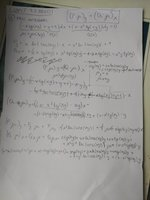# First integral

#### yoscar04

##### Full Member
Hi, I am not sure what your question is. Is this part of a course? Which course? What have you tried?

#### Patricia662

##### New memberThats part of diferential equations. I dont know how to use this function µ.( (P(x)*µ)/dy)=( (Q(x)*µ)/dx). Because ( (P(x)/dy) is not equilavent to ( (Q(x)/dx) you need to.multiple with a function what we call integration multiplicator. And now my question is: is this function actually x*y or what? On the photo is something little i have tried. I understand concept but not with what i need to multiple that P(x) and Q(x) so it will be the same. I hope i wrote it understandable. Thank you

#### joshuaa

##### Junior Member
excellent you found the integration factor

but i will mention an important thing

$$\displaystyle \int -x \tan(xy) \ d(xy) = x \ln|\cos(xy)|$$

here $$\displaystyle x$$ is considered as a constant, so it is ok to ignore it

then, the integration factor is

$$\displaystyle \mu (xy) = e^{\ln|\cos(xy)|} = \cos(xy)$$

now you have

$$\displaystyle M = \cos(xy)(-xy\tan(xy) + y + 1)$$

$$\displaystyle N = \cos(xy)(x - x^2\tan(xy))$$

And you can define a new function $$\displaystyle g(y)$$ as

$$\displaystyle g(y) = \int \left(N - \frac{\partial}{\partial y} \int M \ dx \right) \ dy$$

$$\displaystyle = \int \left(\cos(xy)(x - x^2\tan(xy)) - \frac{\partial}{\partial y} \int \cos(xy)(-xy\tan(xy) + y + 1) \ dx \right) \ dy$$

$$\displaystyle = \int \left(\cos(xy)(x - x^2\tan(xy)) - \frac{\partial}{\partial y} \ \sin(xy) + x \cos(xy) \right) \ dy$$

$$\displaystyle = \int \left(\cos(xy)(x - x^2\tan(xy)) - (x \cos(xy) - x^2 \sin(xy))\right) \ dy$$

$$\displaystyle = \int \left( x\cos(xy) - x^2\sin(xy) - x \cos(xy) + x^2 \sin(xy)\right) \ dy$$

$$\displaystyle = \int 0 \ dy = 0$$

the left side of the equation is

$$\displaystyle f(x, y) = \int M \ dx + g(y)$$

$$\displaystyle = \int \cos(xy)(-xy\tan(xy) + y + 1) \ dx + 0$$

$$\displaystyle = \int -xy\sin(xy) + y\cos(xy) + \cos(xy)) \ dx$$

$$\displaystyle = \sin(xy) + x\cos(xy)$$

the right side of the equation is just a constant

then the final solution is

$$\displaystyle \sin(xy) + x\cos(xy) = C$$